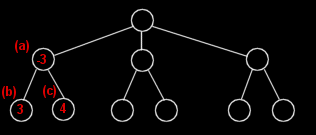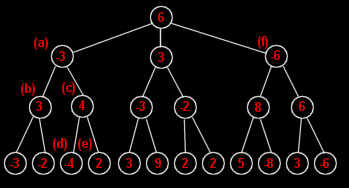### Negamax

Imagine that a position is assigned a value based on its quality from the point of view of the side to move. A positive value would indicate that the side on the move is winning, a negative value that they are losing; a zero value would indicate that neither side has the advantage.

If an evaluation function exists that will provide such a value for any given position then the best move from any position can be assumed to be the move that results in the position with the lowest value (the worst position for the opponent).

Consider the game tree below. Each node represents a game position and each child node represents a position that can result from its parent. The operators in the tree are the legal moves from each position.Figure 1. A game tree of depth 2

If the evaluation function is applied to each of the terminal nodes the value of the non-terminal nodes can be determined by assuming the following

• The value of a position is the value of the best move that can be made from it
• The value of a move is the negative of the value of the position that results from it
In figure 1, the value of -3 at node a is based on the values at nodes b and c. The moves that result in the positions at nodes b and c are valued at -3 and -4 respectively (the negated values of the positions that result from them). Node a is given the value of the best available move from that position (the move that results in the position of node b). Figure 2 shows the procedure applied throughout a game tree of depth 3.Figure 2 The negamax procedure applied to a game tree of depth 3

The best move from the original position is whichever move resulted in the root node being valued at +6. In this case that move was the one that resulted in the position at node f, the worst position for the opponent.

Cookies help us deliver our Services. By using our Services or clicking I agree, you agree to our use of cookies. Learn More.